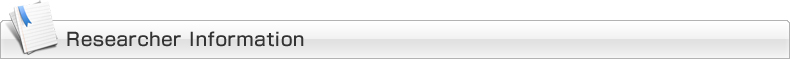##### Faculty, Affiliation

Faculty of Electrical, Information and Communication Engineering,Institute of Science and Engineering
Professor

### College and School Educational Field

Division of Electrical Engineering and Computer Science, Graduate School of Natural Science and Technology
Division of Electrical Engineering and Computer Science, Graduate School of Natural Science and Technology
Course in Information and Communication Engineering, School of Electrical, Information and Communication Engineering, College of Science and Engineering

### Year & Month of Birth

Mathematical Society of Japan

Basic analysis

### Speciality Keywords

population dynamics,Evolution Equations

### Conference Presentations

• Linearized stability for abstract age-structured population equations with delay in Banach spaces(conference：The 6th Asian Conference on Nao-Asia 2018)(2018/11/08)
• Optimal harvesting for size-structured population models with spatial diffusion(conference：Equadiff 2017)(2017/07/24)
• PDE's in Banach lattices and optimal harvesting problems for size-structured population models(conference：Mathematics Seminars)(2016/08/17)
• Partial differential equations in Banach lattices related to size-structured population models(conference：Equadiff2015)(2015/07/06)
• Size-structured population models with diffusion(conference：AIMS2014)(2014/07/07)
• Linearized stability for abstract size-structured population equations with delay(conference：Equadiff 2019)(2019/07/08)

### Classes (Bachelors)

○Theory of Fourier Analysis and Exercise(2017)
○Mathematical Thinking(2017)
○Mathematical Thinking(2017)
○Differential and Integral Calculus 2(2017)
○Differential and Integral Calculus 2(2017)
○Theory of Differential Equations and Exercise(2017)
○Theory of Fourier Analysis and Exercise(2017)
○Theory of Fourier Analysis and Exercise(2017)
○Theory of Fourier Analysis and Exercise(2017)
○Theory of Fourier Analysis and Exercise(2016)
○Theory of Fourier Analysis and Exercise(2016)
○Theory of Fourier Analysis and Exercise(2016)
○Theory of Differential Equations and Exercise(2016)
○Differential and Integral Calculus 2(2016)
○Differential and Integral Calculus 2(2016)
○Mathematical Thinking(2016)

○Introduction to evolution equations(2017)
○Introduction to evolution equations(2017)
○Topics in Mathematical Analysis(2017)
○Introduction to evolution equations(2017)
○Introduction to evolution equations(2017)
○Topics in Mathematical Analysis(2016)
○Introduction to evolution equations(2016)

To Page Top